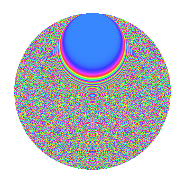# Properties

 Label 1575.2.ekLevel 1575 Weight 2 Character orbit ek Rep. character $$\chi_{1575}(2,\cdot)$$ Character field $$\Q(\zeta_{60})$$ Dimension 3776 Sturm bound 480

# Learn more about

## Defining parameters

 Level: $$N$$ = $$1575 = 3^{2} \cdot 5^{2} \cdot 7$$ Weight: $$k$$ = $$2$$ Character orbit: $$[\chi]$$ = 1575.ek (of order $$60$$ and degree $$16$$) Character conductor: $$\operatorname{cond}(\chi)$$ = $$1575$$ Character field: $$\Q(\zeta_{60})$$ Sturm bound: $$480$$

## Dimensions

The following table gives the dimensions of various subspaces of $$M_{2}(1575, [\chi])$$.

Total New Old
Modular forms 3904 3904 0
Cusp forms 3776 3776 0
Eisenstein series 128 128 0

## Trace form

 $$3776q$$ $$\mathstrut -\mathstrut 24q^{2}$$ $$\mathstrut -\mathstrut 8q^{3}$$ $$\mathstrut +\mathstrut 10q^{4}$$ $$\mathstrut -\mathstrut 24q^{6}$$ $$\mathstrut -\mathstrut 8q^{7}$$ $$\mathstrut -\mathstrut 10q^{9}$$ $$\mathstrut +\mathstrut O(q^{10})$$ $$3776q$$ $$\mathstrut -\mathstrut 24q^{2}$$ $$\mathstrut -\mathstrut 8q^{3}$$ $$\mathstrut +\mathstrut 10q^{4}$$ $$\mathstrut -\mathstrut 24q^{6}$$ $$\mathstrut -\mathstrut 8q^{7}$$ $$\mathstrut -\mathstrut 10q^{9}$$ $$\mathstrut -\mathstrut 16q^{10}$$ $$\mathstrut +\mathstrut 12q^{12}$$ $$\mathstrut -\mathstrut 16q^{13}$$ $$\mathstrut -\mathstrut 30q^{14}$$ $$\mathstrut -\mathstrut 26q^{15}$$ $$\mathstrut -\mathstrut 442q^{16}$$ $$\mathstrut +\mathstrut 18q^{17}$$ $$\mathstrut -\mathstrut 20q^{19}$$ $$\mathstrut -\mathstrut 48q^{20}$$ $$\mathstrut -\mathstrut 12q^{21}$$ $$\mathstrut -\mathstrut 24q^{22}$$ $$\mathstrut -\mathstrut 16q^{25}$$ $$\mathstrut -\mathstrut 8q^{27}$$ $$\mathstrut -\mathstrut 36q^{28}$$ $$\mathstrut -\mathstrut 60q^{29}$$ $$\mathstrut +\mathstrut 10q^{30}$$ $$\mathstrut +\mathstrut 6q^{31}$$ $$\mathstrut +\mathstrut 60q^{32}$$ $$\mathstrut -\mathstrut 42q^{33}$$ $$\mathstrut -\mathstrut 20q^{34}$$ $$\mathstrut -\mathstrut 40q^{36}$$ $$\mathstrut -\mathstrut 16q^{37}$$ $$\mathstrut -\mathstrut 50q^{39}$$ $$\mathstrut +\mathstrut 16q^{40}$$ $$\mathstrut -\mathstrut 36q^{41}$$ $$\mathstrut -\mathstrut 34q^{42}$$ $$\mathstrut -\mathstrut 16q^{43}$$ $$\mathstrut -\mathstrut 102q^{45}$$ $$\mathstrut -\mathstrut 12q^{46}$$ $$\mathstrut -\mathstrut 24q^{47}$$ $$\mathstrut -\mathstrut 98q^{48}$$ $$\mathstrut -\mathstrut 96q^{50}$$ $$\mathstrut -\mathstrut 16q^{51}$$ $$\mathstrut -\mathstrut 48q^{52}$$ $$\mathstrut -\mathstrut 10q^{54}$$ $$\mathstrut -\mathstrut 84q^{55}$$ $$\mathstrut -\mathstrut 18q^{56}$$ $$\mathstrut +\mathstrut 8q^{57}$$ $$\mathstrut -\mathstrut 8q^{58}$$ $$\mathstrut -\mathstrut 30q^{59}$$ $$\mathstrut -\mathstrut 36q^{60}$$ $$\mathstrut +\mathstrut 6q^{61}$$ $$\mathstrut -\mathstrut 134q^{63}$$ $$\mathstrut -\mathstrut 80q^{64}$$ $$\mathstrut +\mathstrut 48q^{65}$$ $$\mathstrut -\mathstrut 30q^{66}$$ $$\mathstrut +\mathstrut 8q^{67}$$ $$\mathstrut -\mathstrut 110q^{69}$$ $$\mathstrut +\mathstrut 32q^{70}$$ $$\mathstrut +\mathstrut 36q^{72}$$ $$\mathstrut -\mathstrut 16q^{73}$$ $$\mathstrut +\mathstrut 96q^{75}$$ $$\mathstrut -\mathstrut 16q^{76}$$ $$\mathstrut -\mathstrut 72q^{77}$$ $$\mathstrut -\mathstrut 154q^{78}$$ $$\mathstrut +\mathstrut 10q^{79}$$ $$\mathstrut -\mathstrut 36q^{80}$$ $$\mathstrut -\mathstrut 6q^{81}$$ $$\mathstrut -\mathstrut 12q^{82}$$ $$\mathstrut -\mathstrut 48q^{83}$$ $$\mathstrut -\mathstrut 260q^{84}$$ $$\mathstrut -\mathstrut 16q^{85}$$ $$\mathstrut +\mathstrut 18q^{87}$$ $$\mathstrut -\mathstrut 32q^{88}$$ $$\mathstrut -\mathstrut 150q^{89}$$ $$\mathstrut -\mathstrut 76q^{90}$$ $$\mathstrut -\mathstrut 24q^{91}$$ $$\mathstrut -\mathstrut 132q^{92}$$ $$\mathstrut -\mathstrut 14q^{93}$$ $$\mathstrut +\mathstrut 10q^{94}$$ $$\mathstrut +\mathstrut 36q^{95}$$ $$\mathstrut -\mathstrut 102q^{96}$$ $$\mathstrut -\mathstrut 16q^{97}$$ $$\mathstrut +\mathstrut 48q^{98}$$ $$\mathstrut +\mathstrut O(q^{100})$$

## Decomposition of $$S_{2}^{\mathrm{new}}(1575, [\chi])$$ into irreducible Hecke orbits

The newforms in this space have not yet been added to the LMFDB.